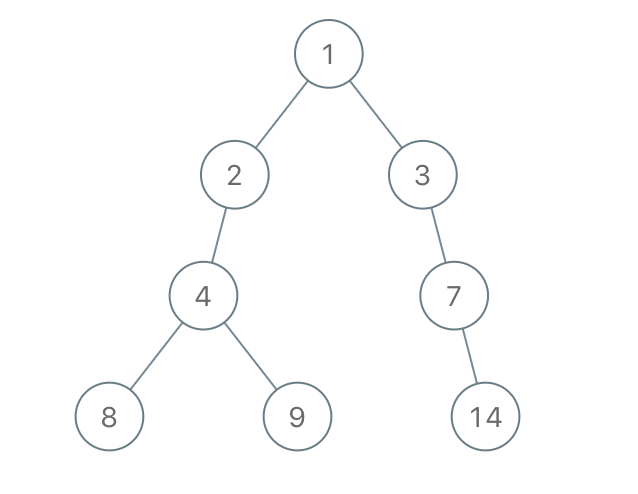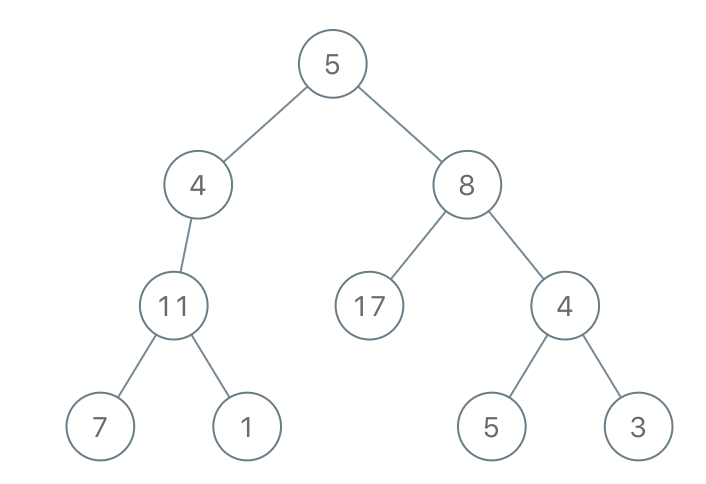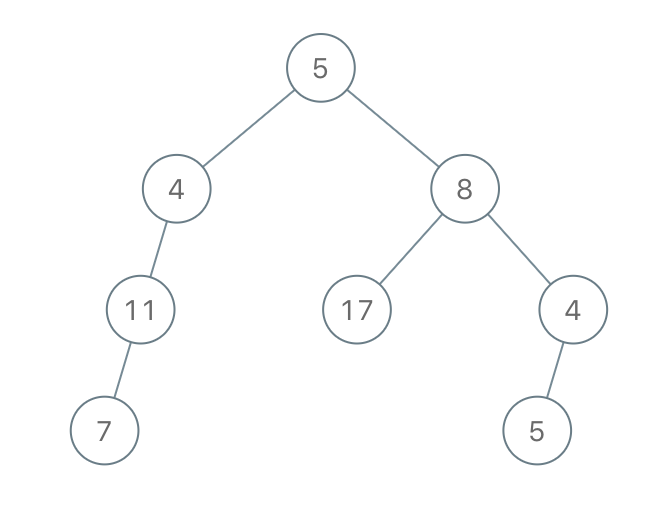Formatted question description: https://leetcode.ca/all/1080.html

# 1080. Insufficient Nodes in Root to Leaf Paths (Medium)

Given the root of a binary tree, consider all root to leaf paths: paths from the root to any leaf.  (A leaf is a node with no children.)

A node is insufficient if every such root to leaf path intersecting this node has sum strictly less than limit.

Delete all insufficient nodes simultaneously, and return the root of the resulting binary tree.

Example 1:Input: root = [1,2,3,4,-99,-99,7,8,9,-99,-99,12,13,-99,14], limit = 1Output: [1,2,3,4,null,null,7,8,9,null,14]


Example 2:Input: root = [5,4,8,11,null,17,4,7,1,null,null,5,3], limit = 22Output: [5,4,8,11,null,17,4,7,null,null,null,5]

Note:

1. The given tree will have between 1 and 5000 nodes.
2. -10^5 <= node.val <= 10^5
3. -10^9 <= limit <= 10^9

## Solution 1.

In order to get the path sum from root to leaf node intersecting with the current node, we need to path sum from root to the current node, and the path sum from the current node to leaf node.

So we can use postorder traversal.

To get the “path sum from root to the current node”, we can pass in the path sum from root to parent as parameter.

To get the “path sum from the current node to leaf node”, we can use the return value of postorder tranversal – returning the maximum path sum from the node to it’s leaf nodes.

// OJ: https://leetcode.com/problems/insufficient-nodes-in-root-to-leaf-paths/

// Time: O(N)
// Space: O(N)
class Solution {
private:
int postorder(TreeNode *root, long long sum, int limit) {
if (!root) return 0;
sum += root->val;
int left = postorder(root->left, sum, limit);
int right = postorder(root->right, sum, limit);
if (sum + left < limit) root->left = NULL;
if (sum + right < limit) root->right = NULL;
return root->val + max(left, right);
}
public:
TreeNode* sufficientSubset(TreeNode* root, int limit) {
return postorder(root, 0, limit) < limit ? NULL : root;
}
};


Java

/**
* Definition for a binary tree node.
* public class TreeNode {
*     int val;
*     TreeNode left;
*     TreeNode right;
*     TreeNode(int x) { val = x; }
* }
*/
class Solution {
public TreeNode sufficientSubset(TreeNode root, int limit) {
boolean rootRemain = depthFirstSearch(root, 0, limit);
return rootRemain ? root : null;
}

private boolean depthFirstSearch(TreeNode node, int sum, int limit) {
if (node.left == null && node.right == null)
return sum + node.val >= limit;
boolean leftRemain = false;
boolean rightRemain = false;
if (node.left != null)
leftRemain = depthFirstSearch(node.left, sum + node.val, limit);
if (node.right != null)
rightRemain = depthFirstSearch(node.right, sum + node.val, limit);
if (!leftRemain)
node.left = null;
if (!rightRemain)
node.right = null;
return leftRemain || rightRemain;
}
}